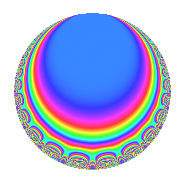Properties

 Label 25.3.c.aLevel 25 Weight 3 Character orbit 25.c Analytic conductor 0.681 Analytic rank 0 Dimension 4 CM No Inner twists 4

Related objects

Newspace parameters

 Level: $$N$$ = $$25 = 5^{2}$$ Weight: $$k$$ = $$3$$ Character orbit: $$[\chi]$$ = 25.c (of order $$4$$ and degree $$2$$)

Newform invariants

 Self dual: No Analytic conductor: $$0.681200660901$$ Analytic rank: $$0$$ Dimension: $$4$$ Relative dimension: $$2$$ over $$\Q(i)$$ Coefficient field: $$\Q(i, \sqrt{6})$$ Coefficient ring: $$\Z[a_1, \ldots, a_{4}]$$ Coefficient ring index: $$1$$ Sato-Tate group: $\mathrm{SU}(2)[C_{4}]$

$q$-expansion

Coefficients of the $$q$$-expansion are expressed in terms of a basis $$1,\beta_1,\beta_2,\beta_3$$ for the coefficient ring described below. We also show the integral $$q$$-expansion of the trace form.

 $$f(q)$$ $$=$$ $$q$$ $$+ \beta_{1} q^{2}$$ $$+ \beta_{3} q^{3}$$ $$-\beta_{2} q^{4}$$ $$-3 q^{6}$$ $$-4 \beta_{1} q^{7}$$ $$-5 \beta_{3} q^{8}$$ $$+ 6 \beta_{2} q^{9}$$ $$+O(q^{10})$$ $$q$$ $$+ \beta_{1} q^{2}$$ $$+ \beta_{3} q^{3}$$ $$-\beta_{2} q^{4}$$ $$-3 q^{6}$$ $$-4 \beta_{1} q^{7}$$ $$-5 \beta_{3} q^{8}$$ $$+ 6 \beta_{2} q^{9}$$ $$-3 q^{11}$$ $$+ \beta_{1} q^{12}$$ $$+ 6 \beta_{3} q^{13}$$ $$-12 \beta_{2} q^{14}$$ $$+ 11 q^{16}$$ $$+ 11 \beta_{1} q^{17}$$ $$+ 6 \beta_{3} q^{18}$$ $$+ 5 \beta_{2} q^{19}$$ $$+ 12 q^{21}$$ $$-3 \beta_{1} q^{22}$$ $$-14 \beta_{3} q^{23}$$ $$+ 15 \beta_{2} q^{24}$$ $$-18 q^{26}$$ $$-15 \beta_{1} q^{27}$$ $$+ 4 \beta_{3} q^{28}$$ $$-30 \beta_{2} q^{29}$$ $$-38 q^{31}$$ $$-9 \beta_{1} q^{32}$$ $$-3 \beta_{3} q^{33}$$ $$+ 33 \beta_{2} q^{34}$$ $$+ 6 q^{36}$$ $$+ 16 \beta_{1} q^{37}$$ $$+ 5 \beta_{3} q^{38}$$ $$-18 \beta_{2} q^{39}$$ $$+ 57 q^{41}$$ $$+ 12 \beta_{1} q^{42}$$ $$-4 \beta_{3} q^{43}$$ $$+ 3 \beta_{2} q^{44}$$ $$+ 42 q^{46}$$ $$+ 6 \beta_{1} q^{47}$$ $$+ 11 \beta_{3} q^{48}$$ $$-\beta_{2} q^{49}$$ $$-33 q^{51}$$ $$+ 6 \beta_{1} q^{52}$$ $$+ 26 \beta_{3} q^{53}$$ $$-45 \beta_{2} q^{54}$$ $$-60 q^{56}$$ $$-5 \beta_{1} q^{57}$$ $$-30 \beta_{3} q^{58}$$ $$+ 90 \beta_{2} q^{59}$$ $$-28 q^{61}$$ $$-38 \beta_{1} q^{62}$$ $$-24 \beta_{3} q^{63}$$ $$-71 \beta_{2} q^{64}$$ $$+ 9 q^{66}$$ $$-39 \beta_{1} q^{67}$$ $$-11 \beta_{3} q^{68}$$ $$+ 42 \beta_{2} q^{69}$$ $$+ 42 q^{71}$$ $$+ 30 \beta_{1} q^{72}$$ $$+ 11 \beta_{3} q^{73}$$ $$+ 48 \beta_{2} q^{74}$$ $$+ 5 q^{76}$$ $$+ 12 \beta_{1} q^{77}$$ $$-18 \beta_{3} q^{78}$$ $$-80 \beta_{2} q^{79}$$ $$-9 q^{81}$$ $$+ 57 \beta_{1} q^{82}$$ $$+ 91 \beta_{3} q^{83}$$ $$-12 \beta_{2} q^{84}$$ $$+ 12 q^{86}$$ $$+ 30 \beta_{1} q^{87}$$ $$+ 15 \beta_{3} q^{88}$$ $$-15 \beta_{2} q^{89}$$ $$+ 72 q^{91}$$ $$-14 \beta_{1} q^{92}$$ $$-38 \beta_{3} q^{93}$$ $$+ 18 \beta_{2} q^{94}$$ $$+ 27 q^{96}$$ $$-44 \beta_{1} q^{97}$$ $$-\beta_{3} q^{98}$$ $$-18 \beta_{2} q^{99}$$ $$+O(q^{100})$$ $$\operatorname{Tr}(f)(q)$$ $$=$$ $$4q$$ $$\mathstrut -\mathstrut 12q^{6}$$ $$\mathstrut +\mathstrut O(q^{10})$$ $$4q$$ $$\mathstrut -\mathstrut 12q^{6}$$ $$\mathstrut -\mathstrut 12q^{11}$$ $$\mathstrut +\mathstrut 44q^{16}$$ $$\mathstrut +\mathstrut 48q^{21}$$ $$\mathstrut -\mathstrut 72q^{26}$$ $$\mathstrut -\mathstrut 152q^{31}$$ $$\mathstrut +\mathstrut 24q^{36}$$ $$\mathstrut +\mathstrut 228q^{41}$$ $$\mathstrut +\mathstrut 168q^{46}$$ $$\mathstrut -\mathstrut 132q^{51}$$ $$\mathstrut -\mathstrut 240q^{56}$$ $$\mathstrut -\mathstrut 112q^{61}$$ $$\mathstrut +\mathstrut 36q^{66}$$ $$\mathstrut +\mathstrut 168q^{71}$$ $$\mathstrut +\mathstrut 20q^{76}$$ $$\mathstrut -\mathstrut 36q^{81}$$ $$\mathstrut +\mathstrut 48q^{86}$$ $$\mathstrut +\mathstrut 288q^{91}$$ $$\mathstrut +\mathstrut 108q^{96}$$ $$\mathstrut +\mathstrut O(q^{100})$$

Basis of coefficient ring in terms of a root $$\nu$$ of $$x^{4}\mathstrut +\mathstrut$$ $$9$$:

 $$\beta_{0}$$ $$=$$ $$1$$ $$\beta_{1}$$ $$=$$ $$\nu$$ $$\beta_{2}$$ $$=$$ $$\nu^{2}$$$$/3$$ $$\beta_{3}$$ $$=$$ $$\nu^{3}$$$$/3$$
 $$1$$ $$=$$ $$\beta_0$$ $$\nu$$ $$=$$ $$\beta_{1}$$ $$\nu^{2}$$ $$=$$ $$3$$ $$\beta_{2}$$ $$\nu^{3}$$ $$=$$ $$3$$ $$\beta_{3}$$

Character Values

We give the values of $$\chi$$ on generators for $$\left(\mathbb{Z}/25\mathbb{Z}\right)^\times$$.

 $$n$$ $$2$$ $$\chi(n)$$ $$\beta_{2}$$

Embeddings

For each embedding $$\iota_m$$ of the coefficient field, the values $$\iota_m(a_n)$$ are shown below.

For more information on an embedded modular form you can click on its label.

Label $$\iota_m(\nu)$$ $$a_{2}$$ $$a_{3}$$ $$a_{4}$$ $$a_{5}$$ $$a_{6}$$ $$a_{7}$$ $$a_{8}$$ $$a_{9}$$ $$a_{10}$$
7.1
 −1.22474 − 1.22474i 1.22474 + 1.22474i −1.22474 + 1.22474i 1.22474 − 1.22474i
−1.22474 1.22474i 1.22474 1.22474i 1.00000i 0 −3.00000 4.89898 + 4.89898i −6.12372 + 6.12372i 6.00000i 0
7.2 1.22474 + 1.22474i −1.22474 + 1.22474i 1.00000i 0 −3.00000 −4.89898 4.89898i 6.12372 6.12372i 6.00000i 0
18.1 −1.22474 + 1.22474i 1.22474 + 1.22474i 1.00000i 0 −3.00000 4.89898 4.89898i −6.12372 6.12372i 6.00000i 0
18.2 1.22474 1.22474i −1.22474 1.22474i 1.00000i 0 −3.00000 −4.89898 + 4.89898i 6.12372 + 6.12372i 6.00000i 0
 $$n$$: e.g. 2-40 or 990-1000 Significant digits: Format: Complex embeddings Normalized embeddings Satake parameters Satake angles

Inner twists

Char. orbit Parity Mult. Self Twist Proved
1.a Even 1 trivial yes
5.b Even 1 yes
5.c Odd 2 yes

Hecke kernels

There are no other newforms in $$S_{3}^{\mathrm{new}}(25, [\chi])$$.